Amalgam

This form shows the cube (h), the dodecahedron (d), the tetrahexahedron (f), and the icositetrahedron…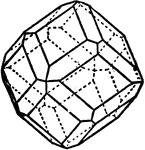Combination of Icositetrahedron and Rhombic Dodecahedron

The combination of icositetrahedron and rhombic dodecahedron.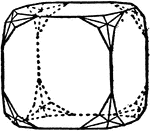Combination of Icositetrahedron and Cube

The combination of an icositetrahedron and a cube.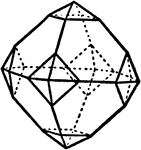Combination of Icosietetrahedron and Octahedron

The combination of an icositetrahedron and an octahedron.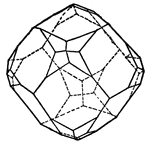Dodecahedron

Crystal; This form is a combination of an icositetrahedron and a rhombic dodecahedron, where the faces…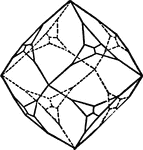Dodecahedron

Crystal; This form is a combination of an icositetrahedron and a rhombic dodecahedron, where the faces…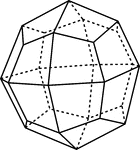Icositetrahedron

"Bounded by twenty-four trapezoidal faces, and hence somethings called a 'trapezohedron.'" -The Encyclopedia…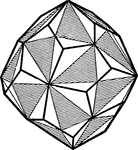Lef-Handed Pentagonal Icositetrahedron

Crystal; If the alternate planes of the hexoctahedron extend until the intersect, a new for will result.…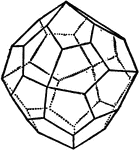Pentagonal Icositetrahedron

"This is the only simple form in this class which differs geometricalled from those of the holosymmetric…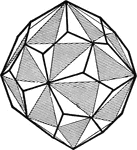Right-Handed Pentagonal Icositetrahedron

Crystal; If the alternate planes of the hexoctahedron extend until the intersect, a new for will result.…